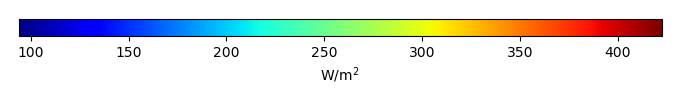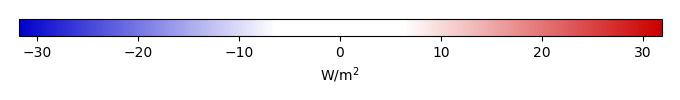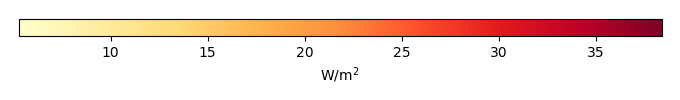# Mean State

Period Mean (original grids) [W/m2]
Model Period Mean (intersection) [W/m2]
Model Period Mean (complement) [W/m2]
Benchmark Period Mean (intersection) [W/m2]
Benchmark Period Mean (complement) [W/m2]
Bias [W/m2]
RMSE [W/m2]
Phase Shift [months]
Bias Score 
RMSE Score 
Seasonal Cycle Score 
Spatial Distribution Score 
Interannual Variability Score 
Overall Score 
Benchmark [-] 401.
CLM5PHSOFF [-] 398. 398. 400. 415. -1.37 9.58 1.81 0.505 0.389 0.734 0.914 0.650 0.597
CLM5PHSON [-] 397. 398. 400. 415. -1.37 9.58 1.81 0.505 0.389 0.734 0.914 0.650 0.597
Period Mean (original grids) [W/m2]
Model Period Mean (intersection) [W/m2]
Model Period Mean (complement) [W/m2]
Benchmark Period Mean (intersection) [W/m2]
Benchmark Period Mean (complement) [W/m2]
Bias [W/m2]
RMSE [W/m2]
Phase Shift [months]
Bias Score 
RMSE Score 
Seasonal Cycle Score 
Spatial Distribution Score 
Interannual Variability Score 
Overall Score 
Benchmark [-] 391.
CLM5PHSOFF [-] 386. 386. 391. 404. -4.87 12.4 0.491 0.729 0.661 0.950 0.915 0.715 0.772
CLM5PHSON [-] 386. 386. 391. 404. -4.87 12.4 0.491 0.729 0.661 0.950 0.915 0.715 0.772
Period Mean (original grids) [W/m2]
Model Period Mean (intersection) [W/m2]
Model Period Mean (complement) [W/m2]
Benchmark Period Mean (intersection) [W/m2]
Benchmark Period Mean (complement) [W/m2]
Bias [W/m2]
RMSE [W/m2]
Phase Shift [months]
Bias Score 
RMSE Score 
Seasonal Cycle Score 
Spatial Distribution Score 
Interannual Variability Score 
Overall Score 
Benchmark [-] 367.
CLM5PHSOFF [-] 363. 363. 366. 384. -2.96 12.2 0.935 0.713 0.624 0.879 0.992 0.747 0.763
CLM5PHSON [-] 361. 361. 366. 384. -2.96 12.2 0.935 0.713 0.624 0.879 0.992 0.747 0.763
Period Mean (original grids) [W/m2]
Model Period Mean (intersection) [W/m2]
Model Period Mean (complement) [W/m2]
Benchmark Period Mean (intersection) [W/m2]
Benchmark Period Mean (complement) [W/m2]
Bias [W/m2]
RMSE [W/m2]
Phase Shift [months]
Bias Score 
RMSE Score 
Seasonal Cycle Score 
Spatial Distribution Score 
Interannual Variability Score 
Overall Score 
Benchmark [-] 243.
CLM5PHSOFF [-] 239. 239. 242. 256. -2.60 13.7 0.123 0.923 0.802 0.992 0.992 0.888 0.900
CLM5PHSON [-] 237. 237. 242. 256. -2.60 13.7 0.123 0.923 0.802 0.992 0.992 0.888 0.900
Period Mean (original grids) [W/m2]
Model Period Mean (intersection) [W/m2]
Model Period Mean (complement) [W/m2]
Benchmark Period Mean (intersection) [W/m2]
Benchmark Period Mean (complement) [W/m2]
Bias [W/m2]
RMSE [W/m2]
Phase Shift [months]
Bias Score 
RMSE Score 
Seasonal Cycle Score 
Spatial Distribution Score 
Interannual Variability Score 
Overall Score 
Benchmark [-] 411.
CLM5PHSOFF [-] 413. 413. 409. 416. 3.75 11.6 1.88 0.366 0.371 0.709 0.567 0.747 0.522
CLM5PHSON [-] 411. 411. 409. 416. 3.75 11.6 1.88 0.366 0.371 0.709 0.567 0.747 0.522
Period Mean (original grids) [W/m2]
Model Period Mean (intersection) [W/m2]
Model Period Mean (complement) [W/m2]
Benchmark Period Mean (intersection) [W/m2]
Benchmark Period Mean (complement) [W/m2]
Bias [W/m2]
RMSE [W/m2]
Phase Shift [months]
Bias Score 
RMSE Score 
Seasonal Cycle Score 
Spatial Distribution Score 
Interannual Variability Score 
Overall Score 
Benchmark [-] 268.
CLM5PHSOFF [-] 265. 265. 267. 280. -2.61 10.9 0.0463 0.917 0.852 0.997 0.974 0.859 0.908
CLM5PHSON [-] 265. 264. 267. 280. -2.61 10.9 0.0463 0.917 0.852 0.997 0.974 0.859 0.908
Period Mean (original grids) [W/m2]
Model Period Mean (intersection) [W/m2]
Model Period Mean (complement) [W/m2]
Benchmark Period Mean (intersection) [W/m2]
Benchmark Period Mean (complement) [W/m2]
Bias [W/m2]
RMSE [W/m2]
Phase Shift [months]
Bias Score 
RMSE Score 
Seasonal Cycle Score 
Spatial Distribution Score 
Interannual Variability Score 
Overall Score 
Benchmark [-] 297.
CLM5PHSOFF [-] 296. 295. 296. 341. -0.587 14.2 0.144 0.843 0.810 0.990 0.987 0.795 0.872
CLM5PHSON [-] 293. 292. 296. 341. -0.587 14.2 0.144 0.843 0.810 0.990 0.987 0.795 0.872
Period Mean (original grids) [W/m2]
Model Period Mean (intersection) [W/m2]
Model Period Mean (complement) [W/m2]
Benchmark Period Mean (intersection) [W/m2]
Benchmark Period Mean (complement) [W/m2]
Bias [W/m2]
RMSE [W/m2]
Phase Shift [months]
Bias Score 
RMSE Score 
Seasonal Cycle Score 
Spatial Distribution Score 
Interannual Variability Score 
Overall Score 
Benchmark [-] 387.
CLM5PHSOFF [-] 379. 379. 385. 415. -5.63 15.4 0.408 0.718 0.713 0.963 0.998 0.799 0.817
CLM5PHSON [-] 374. 375. 385. 415. -5.63 15.4 0.408 0.718 0.713 0.963 0.998 0.799 0.817
Period Mean (original grids) [W/m2]
Model Period Mean (intersection) [W/m2]
Model Period Mean (complement) [W/m2]
Benchmark Period Mean (intersection) [W/m2]
Benchmark Period Mean (complement) [W/m2]
Bias [W/m2]
RMSE [W/m2]
Phase Shift [months]
Bias Score 
RMSE Score 
Seasonal Cycle Score 
Spatial Distribution Score 
Interannual Variability Score 
Overall Score 
Benchmark [-] 249.
CLM5PHSOFF [-] 244. 244. 248. 253. -4.14 13.6 0.0505 0.917 0.797 0.997 0.977 0.914 0.900
CLM5PHSON [-] 243. 244. 248. 253. -4.14 13.6 0.0505 0.917 0.797 0.997 0.977 0.914 0.900
Period Mean (original grids) [W/m2]
Model Period Mean (intersection) [W/m2]
Model Period Mean (complement) [W/m2]
Benchmark Period Mean (intersection) [W/m2]
Benchmark Period Mean (complement) [W/m2]
Bias [W/m2]
RMSE [W/m2]
Phase Shift [months]
Bias Score 
RMSE Score 
Seasonal Cycle Score 
Spatial Distribution Score 
Interannual Variability Score 
Overall Score 
Benchmark [-] 353.
CLM5PHSOFF [-] 351. 351. 352. 359. -0.968 9.75 0.299 0.865 0.762 0.980 0.992 0.867 0.871
CLM5PHSON [-] 351. 351. 352. 359. -0.968 9.75 0.299 0.865 0.762 0.980 0.992 0.867 0.871
Period Mean (original grids) [W/m2]
Model Period Mean (intersection) [W/m2]
Model Period Mean (complement) [W/m2]
Benchmark Period Mean (intersection) [W/m2]
Benchmark Period Mean (complement) [W/m2]
Bias [W/m2]
RMSE [W/m2]
Phase Shift [months]
Bias Score 
RMSE Score 
Seasonal Cycle Score 
Spatial Distribution Score 
Interannual Variability Score 
Overall Score 
Benchmark [-] 314.
CLM5PHSOFF [-] 309. 309. 312. 329. -3.25 11.6 0.223 0.807 0.752 0.982 0.997 0.696 0.831
CLM5PHSON [-] 306. 306. 312. 329. -3.25 11.6 0.223 0.807 0.752 0.982 0.997 0.696 0.831
Period Mean (original grids) [W/m2]
Model Period Mean (intersection) [W/m2]
Model Period Mean (complement) [W/m2]
Benchmark Period Mean (intersection) [W/m2]
Benchmark Period Mean (complement) [W/m2]
Bias [W/m2]
RMSE [W/m2]
Phase Shift [months]
Bias Score 
RMSE Score 
Seasonal Cycle Score 
Spatial Distribution Score 
Interannual Variability Score 
Overall Score 
Benchmark [-] 345.
CLM5PHSOFF [-] 313. 313. 315. 360. -2.77 13.1 0.509 0.757 0.685 0.941 0.999 0.796 0.811
CLM5PHSON [-] 310. 310. 315. 360. -2.77 13.1 0.509 0.757 0.685 0.941 0.999 0.796 0.811
Period Mean (original grids) [W/m2]
Model Period Mean (intersection) [W/m2]
Model Period Mean (complement) [W/m2]
Benchmark Period Mean (intersection) [W/m2]
Benchmark Period Mean (complement) [W/m2]
Bias [W/m2]
RMSE [W/m2]
Phase Shift [months]
Bias Score 
RMSE Score 
Seasonal Cycle Score 
Spatial Distribution Score 
Interannual Variability Score 
Overall Score 
Benchmark [-] 350.
CLM5PHSOFF [-] 345. 345. 349. 373. -3.78 14.4 0.431 0.818 0.734 0.963 0.989 0.789 0.838
CLM5PHSON [-] 344. 344. 349. 373. -3.78 14.4 0.431 0.818 0.734 0.963 0.989 0.789 0.838
Period Mean (original grids) [W/m2]
Model Period Mean (intersection) [W/m2]
Model Period Mean (complement) [W/m2]
Benchmark Period Mean (intersection) [W/m2]
Benchmark Period Mean (complement) [W/m2]
Bias [W/m2]
RMSE [W/m2]
Phase Shift [months]
Bias Score 
RMSE Score 
Seasonal Cycle Score 
Spatial Distribution Score 
Interannual Variability Score 
Overall Score 
Benchmark [-] 248.
CLM5PHSOFF [-] 243. 243. 247. 258. -4.19 13.4 0.126 0.901 0.793 0.992 1.00 0.895 0.896
CLM5PHSON [-] 243. 243. 247. 258. -4.19 13.4 0.126 0.901 0.793 0.992 1.00 0.895 0.896
Period Mean (original grids) [W/m2]
Model Period Mean (intersection) [W/m2]
Model Period Mean (complement) [W/m2]
Benchmark Period Mean (intersection) [W/m2]
Benchmark Period Mean (complement) [W/m2]
Bias [W/m2]
RMSE [W/m2]
Phase Shift [months]
Bias Score 
RMSE Score 
Seasonal Cycle Score 
Spatial Distribution Score 
Interannual Variability Score 
Overall Score 
Benchmark [-] 376.
CLM5PHSOFF [-] 370. 370. 371. 402. -1.23 14.5 0.704 0.640 0.676 0.911 0.994 0.783 0.780
CLM5PHSON [-] 363. 363. 371. 402. -1.23 14.5 0.704 0.640 0.676 0.911 0.994 0.783 0.780
Period Mean (original grids) [W/m2]
Model Period Mean (intersection) [W/m2]
Model Period Mean (complement) [W/m2]
Benchmark Period Mean (intersection) [W/m2]
Benchmark Period Mean (complement) [W/m2]
Bias [W/m2]
RMSE [W/m2]
Phase Shift [months]
Bias Score 
RMSE Score 
Seasonal Cycle Score 
Spatial Distribution Score 
Interannual Variability Score 
Overall Score 
Benchmark [-] 335.
CLM5PHSOFF [-] 331. 331. 333. 372. -2.37 9.63 0.122 0.919 0.831 0.992 1.00 0.814 0.898
CLM5PHSON [-] 328. 328. 333. 372. -2.37 9.64 0.122 0.919 0.831 0.992 1.00 0.814 0.898
Period Mean (original grids) [W/m2]
Model Period Mean (intersection) [W/m2]
Model Period Mean (complement) [W/m2]
Benchmark Period Mean (intersection) [W/m2]
Benchmark Period Mean (complement) [W/m2]
Bias [W/m2]
RMSE [W/m2]
Phase Shift [months]
Bias Score 
RMSE Score 
Seasonal Cycle Score 
Spatial Distribution Score 
Interannual Variability Score 
Overall Score 
Benchmark [-] 278.
CLM5PHSOFF [-] 276. 276. 277. 303. -0.971 9.62 0.0673 0.909 0.823 0.995 0.995 0.864 0.901
CLM5PHSON [-] 275. 275. 277. 303. -0.971 9.62 0.0673 0.909 0.823 0.995 0.995 0.864 0.901
Period Mean (original grids) [W/m2]
Model Period Mean (intersection) [W/m2]
Model Period Mean (complement) [W/m2]
Benchmark Period Mean (intersection) [W/m2]
Benchmark Period Mean (complement) [W/m2]
Bias [W/m2]
RMSE [W/m2]
Phase Shift [months]
Bias Score 
RMSE Score 
Seasonal Cycle Score 
Spatial Distribution Score 
Interannual Variability Score 
Overall Score 
Benchmark [-] 298.
CLM5PHSOFF [-] 294. 295. 298. 325. -3.00 12.5 0.124 0.828 0.763 0.992 0.992 0.738 0.846
CLM5PHSON [-] 293. 294. 298. 325. -3.00 12.5 0.124 0.828 0.763 0.992 0.992 0.738 0.846
Period Mean (original grids) [W/m2]
Model Period Mean (intersection) [W/m2]
Model Period Mean (complement) [W/m2]
Benchmark Period Mean (intersection) [W/m2]
Benchmark Period Mean (complement) [W/m2]
Bias [W/m2]
RMSE [W/m2]
Phase Shift [months]
Bias Score 
RMSE Score 
Seasonal Cycle Score 
Spatial Distribution Score 
Interannual Variability Score 
Overall Score 
Benchmark [-] 410.
CLM5PHSOFF [-] 406. 407. 410. 415. -2.98 10.8 1.26 0.546 0.467 0.837 0.978 0.774 0.678
CLM5PHSON [-] 406. 406. 410. 415. -2.98 10.8 1.26 0.546 0.467 0.837 0.978 0.774 0.678
Period Mean (original grids) [W/m2]
Model Period Mean (intersection) [W/m2]
Model Period Mean (complement) [W/m2]
Benchmark Period Mean (intersection) [W/m2]
Benchmark Period Mean (complement) [W/m2]
Bias [W/m2]
RMSE [W/m2]
Phase Shift [months]
Bias Score 
RMSE Score 
Seasonal Cycle Score 
Spatial Distribution Score 
Interannual Variability Score 
Overall Score 
Benchmark [-] 379.
CLM5PHSOFF [-] 377. 376. 380. 374. -2.98 11.2 0.680 0.765 0.650 0.935 0.994 0.845 0.807
CLM5PHSON [-] 377. 376. 380. 374. -2.98 11.2 0.682 0.765 0.650 0.935 0.994 0.845 0.807
Period Mean (original grids) [W/m2]
Model Period Mean (intersection) [W/m2]
Model Period Mean (complement) [W/m2]
Benchmark Period Mean (intersection) [W/m2]
Benchmark Period Mean (complement) [W/m2]
Bias [W/m2]
RMSE [W/m2]
Phase Shift [months]
Bias Score 
RMSE Score 
Seasonal Cycle Score 
Spatial Distribution Score 
Interannual Variability Score 
Overall Score 
Benchmark [-] 303.
CLM5PHSOFF [-] 305. 304. 298. 346. 6.58 22.1 0.556 0.480 0.531 0.953 0.929 0.746 0.695
CLM5PHSON [-] 301. 301. 298. 346. 6.58 22.1 0.556 0.480 0.531 0.953 0.929 0.746 0.695

# Temporally integrated period mean

BENCHMARK MEANMODEL MEANBIASBIAS SCORERMSERMSE SCOREBENCHMARK INTERANNUAL VARIABILITYMODEL INTERANNUAL VARIABILITYINTERANNUAL VARIABILITY SCOREBENCHMARK MAX MONTHMODEL MAX MONTHDIFFERENCE IN MAX MONTHSEASONAL CYCLE SCORESPATIAL TAYLOR DIAGRAMMODEL COLORS# Spatially integrated regional mean

MODEL COLORSREGIONAL MEANANNUAL CYCLEMONTHLY ANOMALYANNUAL CYCLE# All Models

BenchmarkCLM5PHSOFFCLM5PHSON# Data Information

creation_date: Thu May 8 23:18:00 PDT 2014

source_file: This product is generated from monthly 1 degree CERES EBAF Radiation observations